# 前言

KMP算法是我们数据结构串中最难也是最重要的算法。难是因为KMP算法的代码很优美简洁干练，但里面包含着非常深的思维。真正理解代码的人可以说对KMP算法的了解已经相当深入了。而且这个算法的不少东西的确不容易讲懂，很多正规的书本把概念一摆出直接劝退无数人。这篇文章将尽量以最详细的方式配图介绍KMP算法及其改进。文章的开始我先对KMP算法的三位创始人Knuth,Morris,Pratt致敬，懂得这个算法的流程后你真的不得不佩服他们的聪明才智。

# KMP解决的问题类型

KMP算法的作用是在一个已知字符串中查找子串的位置,也叫做串的模式匹配。比如主串s=“university”,子串t=“sit”。现在我们要找到子串t 在主串s 中的位置。大家肯定觉得这还不简单，不就在第七个嘛，一眼就看出来了。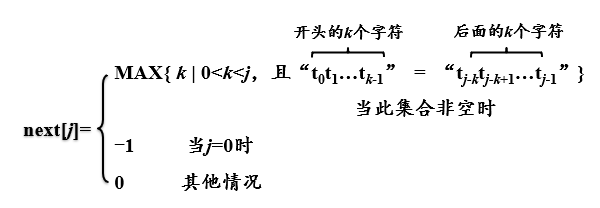# 图解KMP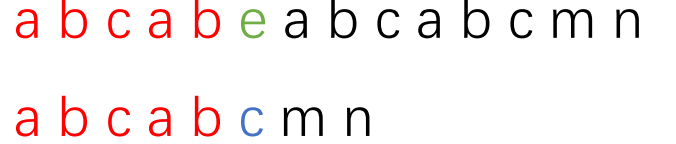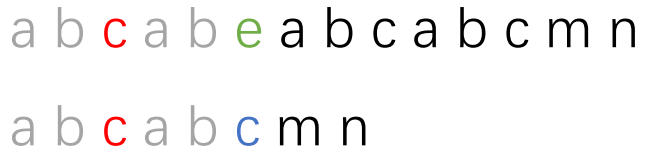next=0；next=0；next=0；next=1；next=2；next=3；next=0；
| a | b |c |a |b | c | m |n |
|–|–|–|–|–|–|–|–|–|–|
|next |next | next | next |next |next | next | next |
|-1 | 0 |0 | 0 | 1 | 2 |3 | 0 |next[i]的值表示下标为i的字符前的字符串最长相等前后缀的长度。

next有这两个作用的源头是：之前提到的字符串的最长相等前后缀

# KMP算法的时间复杂度

KMP算法中多了一个求数组的过程，多消耗了一点点空间。我们设主串s长度为n,子串t的长度为m。求next数组时时间复杂度为O(m)，因后面匹配中主串不回溯，比较次数可记为n，所以KMP算法的总时间复杂度为O(m+n)，空间复杂度记为O(m)。相比于朴素的模式匹配时间复杂度O(m*n)，KMP算法提速是非常大的，这一点点空间消耗换得极高的时间提速是非常有意义的，这种思想也是很重要的。

# 求next数组的代码

``````typedef struct
{
char data[MaxSize];
int length;			//串长
} SqString;
//SqString 是串的数据结构
//typedef重命名结构体变量，可以用SqString t定义一个结构体。
void GetNext(SqString t,int next[])		//由模式串t求出next值
{
int j,k;
j=0;k=-1;
next=-1;//第一个字符前无字符串，给值-1
while (j<t.length-1)
//因为next数组中j最大为t.length-1,而每一步next数组赋值都是在j++之后
//所以最后一次经过while循环时j为t.length-2
{
if (k==-1 || t.data[j]==t.data[k]) 	//k为-1或比较的字符相等时
{
j++;k++;
next[j]=k;
//对应字符匹配情况下，s与t指向同步后移
//通过字符串"aaaaab"求next数组过程想一下这一步的意义
//printf("(1) j=%d,k=%d,next[%d]=%d\n",j,k,j,k);
}
else
{
k=next[k];
**//我们现在知道next[k]的值代表的是下标为k的字符前面的字符串最长相等前后缀的长度
//也表示该处字符不匹配时应该回溯到的字符的下标
//这个值给k后又进行while循环判断，此时t.data[k]即指最长相等前缀后一个字符**
//为什么要回退此处进行比较，我们往下接着看。其实原理和上面介绍的KMP原理差不多
//printf("(2) k=%d\n",k);
}
}
}
``````

## 解释next数组构造过程中的回溯问题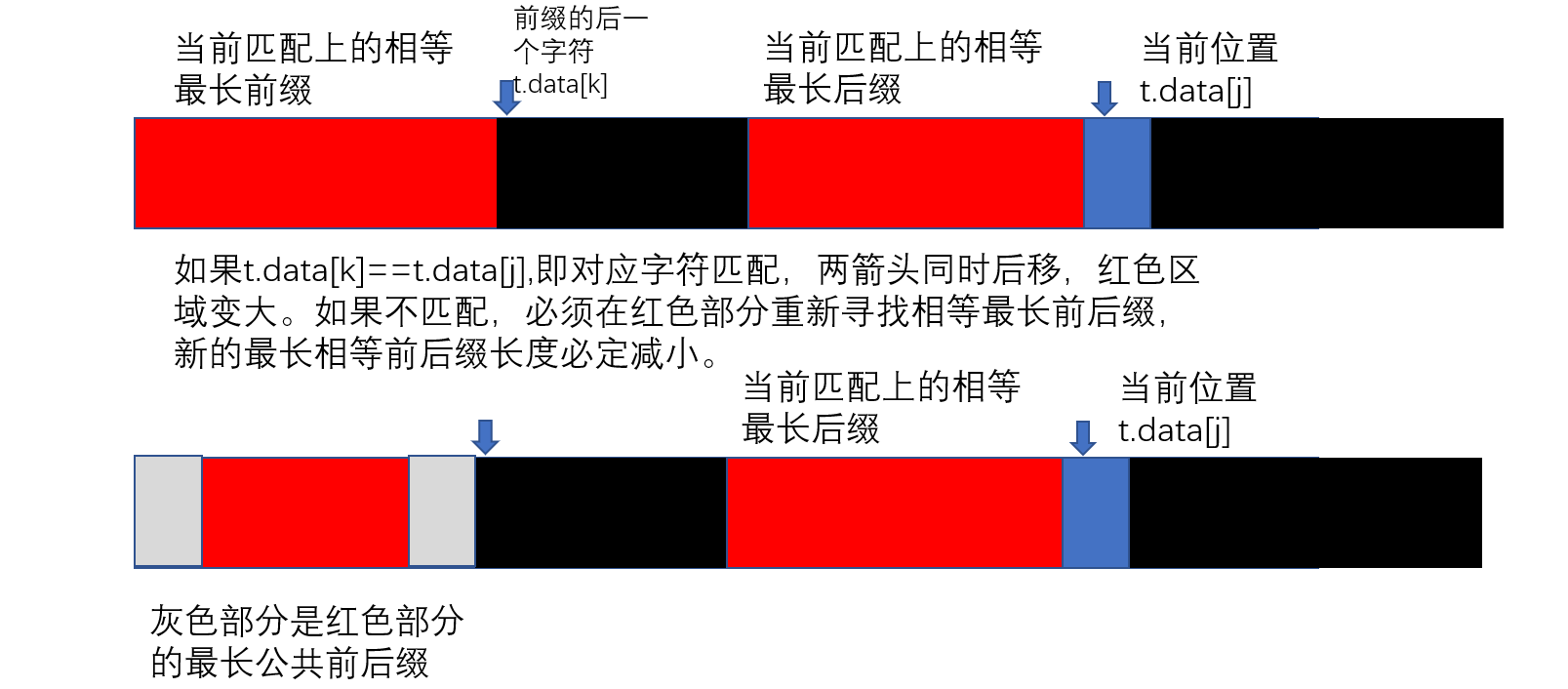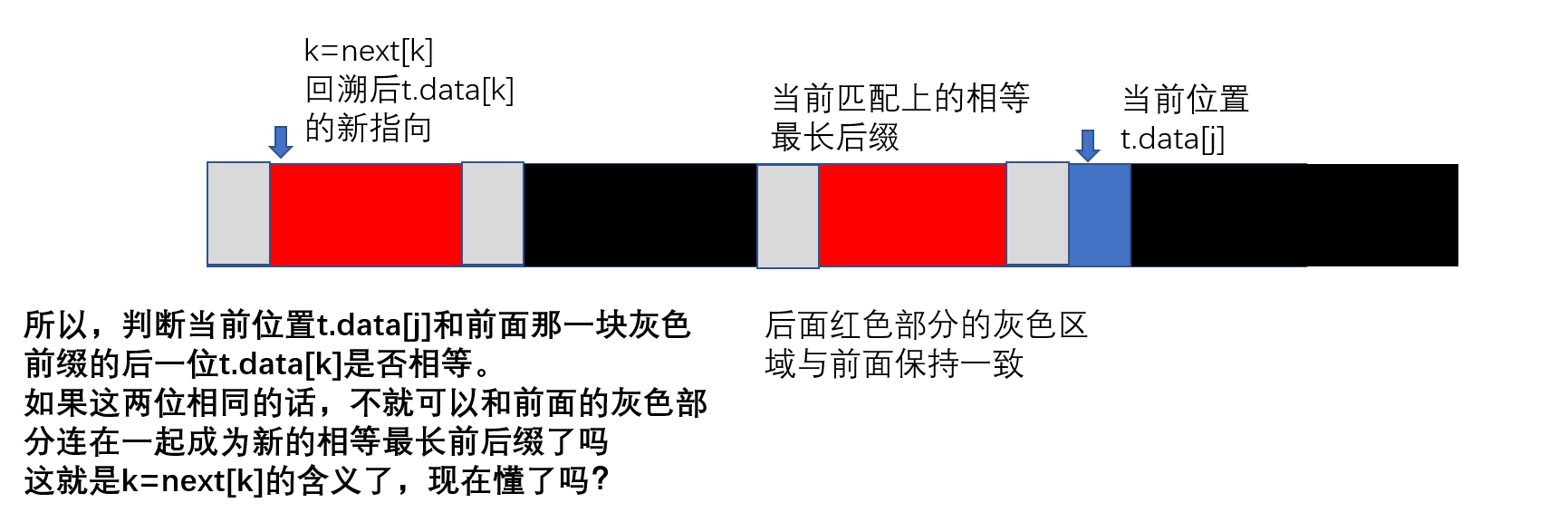# KMP算法代码解释

``````int KMPIndex(SqString s,SqString t)  //KMP算法
{

int next[MaxSize],i=0,j=0;
GetNext(t,next);
while (i<s.length && j<t.length)
{
if (j==-1 || s.data[i]==t.data[j])
{
i++;j++;  			//i,j各增1
}
else j=next[j]; 		//i不变,j后退，现在知道为什么这样让子串回退了吧
}
if (j>=t.length)
return(i-t.length);  	//返回匹配模式串的首字符下标
else
return(-1);        		//返回不匹配标志
}
``````

# KMP算法的改进

| 下标 |0|1|2|3|4|5|6|7
|–|–|–|–|–|–|–|–|–|–|
| 子串 | a | b |a |b |a | a | a | b |
|next |-1 | 0 | 0 |1 |2 | 3 | 1 |1
|nextval | -1 |0 | -1 | 0 |-1 | 3 | 1|0

``````void GetNextval(SqString t,int nextval[])
//由模式串t求出nextval值
{
int j=0,k=-1;
nextval=-1;
while (j<t.length)
{
if (k==-1 || t.data[j]==t.data[k])
{
j++;k++;
if (t.data[j]!=t.data[k])
//这里的t.data[k]是t.data[j]处字符不匹配而会回溯到的字符
//为什么？因为没有这处if判断的话，此处代码是next[j]=k;
//next[j]不就是t.data[j]不匹配时应该回溯到的字符位置嘛
nextval[j]=k;
else
nextval[j]=nextval[k];
//这一个代码含义是不是呼之欲出了？
//此时nextval[j]的值就是就是t.data[j]不匹配时应该回溯到的字符的nextval值
//用较为粗鄙语言表诉：即字符不匹配时回溯两层后对应的字符下标
}
else  k=nextval[k];
}

}
``````
``````int KMPIndex1(SqString s,SqString t)
//修正的KMP算法
//只是next换成了nextval
{
int nextval[MaxSize],i=0,j=0;
GetNextval(t,nextval);
while (i<s.length && j<t.length)
{
if (j==-1 || s.data[i]==t.data[j])
{
i++;j++;
}
else j=nextval[j];
}
if (j>=t.length)
return(i-t.length);
else
return(-1);
}
``````

# 参考书籍

《数据结构教程》（第5版） 李春葆
《大话数据结构》 程杰